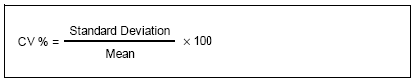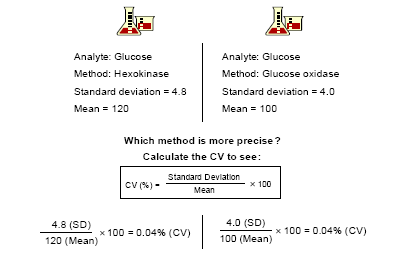##### Coefficient of Variation (CV)

The coefficient of variation is the ratio of the standard deviation to the mean. Express CV as a percentage. Use the following formula to calculate the CV:Using the CV makes it easier to compare the overall precision of two analytical systems. The CV is a more accurate comparison than the standard deviation as the standard deviation typically increases as the concentration of the analyte increases. Comparing precision for two different methods using only the standard deviation can be misleading.

#### Example Scenario:

Compare a hexokinase method and glucose oxidase method for measuring glucose. The standard deviation for the hexokinase method is 4.8. The standard deviation for the glucose oxidase method is 4.0. Based on the standard deviation, you might conclude the glucose oxidase method is more precise than the hexokinase method.

However, in this example, a comparison of the CV shows the methods are equally precise. Assuming the mean for the hexokinase method is 120 and the mean for the glucose oxidase method is 100, the CV for both methods is 4%.

Example of Coefficient of Variation (CV) versus Standard Deviation (SD)In this example, the CV proves both methods are equally precise.